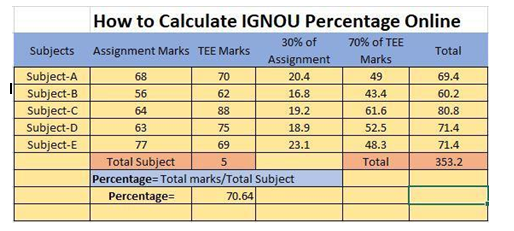# HOW TO CALCULATE IGNOU PERCENTAGE?

Indira Gandhi National Open University (IGNOU) stands as one of the topmost distance and open education establishments in India. It is abbreviated as IGNOU and is located in New Delhi. Different kinds of graduate and post-graduate courses are taught in this institute. At the end of the academic sessions, IGNOU announces its results. But many of the reports state that students of IGNOU often tend to confusion about calculating the IGNOU percentage. This article is an initiative to help you calculate the IGNOU percentage of the end of term exam online.

## Information related to the calculation of percentage.

The percentage of IGNOU for every subject is based on a collective score of assignment and theory. It is important for the students studying in IGNOU to score good marks in both assignment and theory to get a good score overall. TEE exams that are IGNOU’s half-yearly exams are conducted twice a year in December and June. It is required by the students to submit the assignments to the registered IGNOU centers before the last date.

The assignments are given to the students according to their preferred subjects. Every answer should be answered according to the question asked. In any case, if a student copies the assignment answers from any existing solved answers or study material, then the board of IGNOU will not accept the solved assignment papers of the students.

The percentage of IGNOU is calculated based on 30:70 on the subject. The 30:70 ratio stands as-30% assignment marks and 70% theory marks, in a particular subject. It is required by a student who studies in IGNOU to obtain a minimum of 40 out of 100, 20 out of 50 in theory, accompanied by 50 out of 100 in assignment for the semester exam. If there is a case when a student has failed in the theory exam but obtained 50% in an assignment, then students need not submit the assignment paper to the regional center of IGNOU.

Read more: IGNOU – The People’s University

## IGNOU Percentage Calculator

The grading system of IGNOU is based on two categories- achievement of a student in the field of education and discipline. There are various categories of students based on their scores: the students who have a score of 80% and above fall in the category of excellent, 79%-60% score of students stand very good, 59%-50% score students fall in the category of good, the students scoring 49%-40% are satisfactory and finally below 40% appear to be unsatisfactory in the academic grade card. The grading system in IGNOU is devoid of decimal numbers.

The decimals given in the exams are rounded to the nearest whole number. For example, the students who scored 25.1, 25.2, 25.3, 25.4, 25.5 in any subject, then the score presented on the grade card after rounding off will be 25. Similarly, students who score 27.6, 27.7, 27.8, 27.9 5 in any subject, then the score presented on the grade card after rounding off will be 29.

To know about the criteria for calculating the IGNOU percentage, some things should be known to the student calculating the percentage. The total number of marks got in the exam/number of subjects will give the result of the percentage.

## Steps to calculate the percentage

It is a simple procedure to calculate your IGNOU percentage on your own without the help of any internet connection. The main point is that a student should have their accurate theory and assignment marks for all subjects in their respective semesters. IGNOU issues the assignment and theory mark out of 100. There is a need for the students to convert their theory and assignment marks in the percentage ratio of 30:70.

Listed below are some steps that would explain HOW TO CALCULATE IGNOU PERCENTAGE.

Step 1– The student has to collect all the subject’s theory and assignment marks.

Step 2– Make a table and fill up the respective marks for the individual subjects as mentioned below in the table.

Step 3– Convert the assignment and theory marks in the ratio of 30:70. Convert the theory marks into 70% and assignment marks into 30%.

Step 4– Add 70% of the theory marks and 30% of assignment marks to the aggregation of marks.

Step 5– Now sum all the marks of the individual subjects and get a total of all the subjects.

Step 6– Lastly divide the total marks of all the subjects and divide it by the total number of subjects.

Step 7– The final marks that you get are your IGNOU percentage of that academic exams.The above-mentioned table gives a figurative explanation of how to calculate the IGNOU percentage.

• The subject column represents various subjects.

• The assignment Marks column represents the marks a student has obtained in the assignments out of 100.

• The TEE Marks column represents the marks a student has obtained in the theory exams out of 100.

• The 30% of the assignment marks column represents the marks a student has obtained in the assignments out of 30.

• The 70% of the TEE marks column represents the marks a student has obtained in the theory exams out of 70.

• The total column represents the sum of marks obtained in 30% of the assignment marks column and 70% of the TEE marks column.

I hope this article was helpful to every reader in knowing the facts related to the procedure involved in calculating the percentage of IGNOU marks.